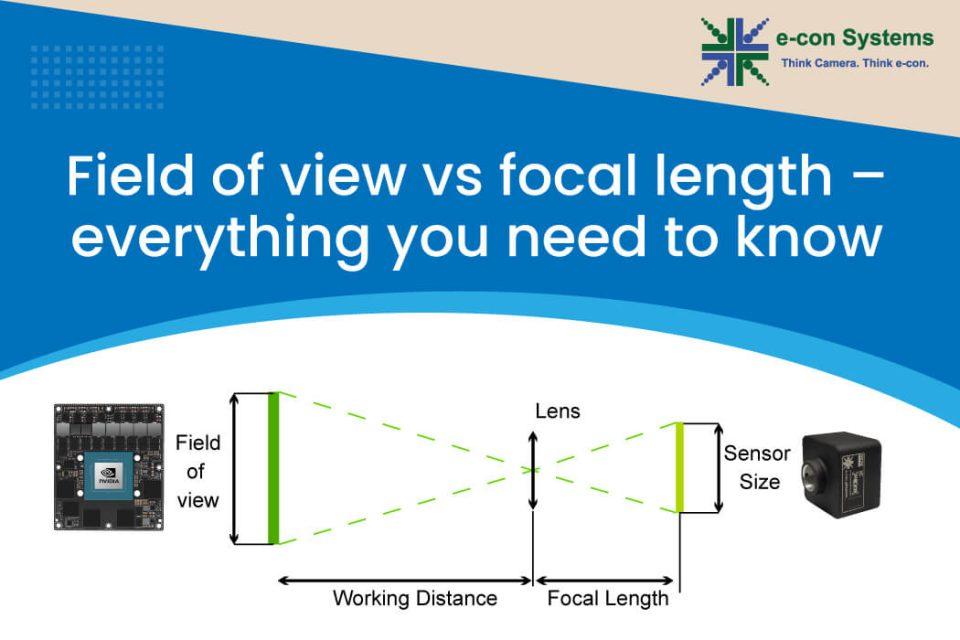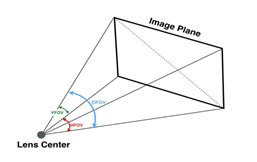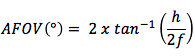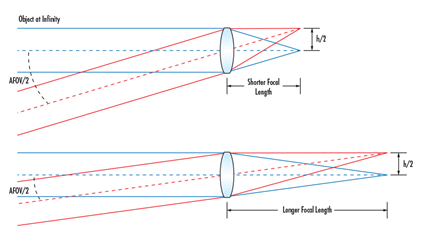# Field of view vs focal length – everything you need to know

## Field of view and focal length are two of the most important lens parameters. As simple as they are, not many understand them thoroughly. Learn what they mean, how the two are related, and why it is important to understand the two concepts thoroughly.Field of view and focal length are two of the most important concepts when it comes to lenses. While focal length is the defining property of a lens, field of view can vary depending on certain other parameters. And when you select a lens for your embedded vision application, you need to make sure that you pick the right one for your sensor such that the desired field of view is achieved.

In this article, we attempt to learn what focal length and field of view are, their differences, and why it is important to understand the two concepts thoroughly when it comes to choosing a lens for your embedded vision application.

### What is field of view?

Field Of View is the maximum area of a scene that a camera can focus on/capture. It is represented in degrees. Depending on how you measure it, FOV can be represented either vertically, horizontally, or diagonally as shown in the image below:Figure 1: Different types of FOV

### How to calculate FOV

FOV of a lens is calculated using the below formula:Where,

AFOV – Angular Field of View

HFOV – Width of the object seen through the lens

Working distance (WD) – Distance between the lens and the object

However, in most cases, the FOV of a lens is expressed using DFOV or Diagonal Field of View. So, you might have to calculate the DFOV value as well. Let us see how that is done.

Usually, the horizontal dimension (which is nothing but the HFOV) and the working distance are given values. Using these, you would be able to calculate AFOV.

Once this is done, invert the formula used to calculate AFOV as given below:Now DFOV can be calculated by replacing HFOV with it in the above equation. Since AFOV and working distance are known entities, DFOV can be derived using this.

Similarly, VFOV or Vertical Field Of View can be calculated by replacing HFOV with the VFOV value in the same equation.

### What is focal length?

Now let’s come to focal length

Focal length is the defining property of a lens. It is the distance between the lens and the plane of the sensor, and is determined when the lens focuses the object at infinity. It is usually represented in millimeters. Its value depends on the curvature and the material of the lens.

### Field of view and focal length – how they are related

The following equation illustrates the relationship between field of view and focal length:Where f is the focal length of the lens and h is the sensor size.

From this equation, it can be understood that the shorter the focal length, the wider the AFOV, and vice versa. This is clearly depicted in the figure below:Figure 2: Focal Length and AFOV

### The significance of understanding the relationship between field of view and focal length

In embedded vision – in most cases – the image sensor is chosen first. This would mean that the choice of lens is heavily determined by the sensor you use (since AFOV depends on the sensor size). For a given sensor size, to achieve a wider FOV, you need to go with a short focal length lens and vice versa. However, since the focal length cannot be made shorter beyond a point, increasing the sensor size also helps to achieve a wider FOV.

### Choosing the right lens for your embedded vision application

Picking the right lens considering multiple factors can sometimes be overwhelming. And this is where e-con Systems can help. While integrating our camera modules, we work closely with our customers to help them choose the best-fit lens for their application. We also extend lens fixation and lens mount customization services.

To learn everything about choosing the right lens for your embedded vision system, please visit the article How to choose the right lens for your embedded camera application.